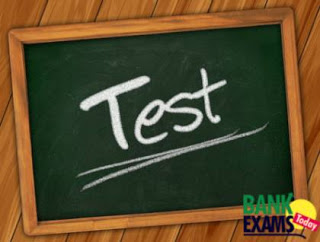New Students Offer - Use Code HELLO

# Mixed Aptitude Quiz -2 ( IBPS PO Pre Exam 2016)#### Ques 1.

Two Trains namely A and B start simultaneously from a certain place at the speed of 40 kmph and 55 kmph, respectively.The train B reaches the destination 2 hours earlier than A. What is the distance between the starting point and destination?

Solution :

Here distance is equal

==> 40*time = 55(time-2)
==>Time = 22/3 hours

Distance = 40*22/3= 294 km approx

#### Ques 2.

The income of Siva and Ganesh are in the ratio of 4:5 and their expenditure is in the ratio of 2:3. If each of them saves 2000, then find their income.

Solution :

Income ratio = 4x : 5x
Expenditure ratio = 2y : 3y
Savings = 2000

==> 4x-2y = 5x-3y
==> x=y    ---- (i)
From eq (i)
==> 4x-2(x)=2000
==> x =1000
Incomes = 4*1000 ; 5*1000 = 4000 ; 5000

#### Ques 3.

A dealer sold two mobiles at Rs. 5940 each. On selling one AC he gained 10% and on selling the other he lost 10%. Find the dealer’s gain or loss percent?

Solution :

Mobile1 sold at 10% profit
110% -------- 5940
100% --------   ?

Mobile 1 cost price = 5400

Mobile 2 sold at 10% loss

90% ----- 5940
100% ----  ?

Mobile 2 cost price = 6600

Total cost price = 6600+5400=12000

Total selling price = 5940*2 = 11880

Profit percentage = (120*100)/12000=1%(1%loss)

#### Ques 4.

A basket contains 5 red 4 blue 3 green balls. If three marbles picked up random, What is the probability that either all are green or all are red?

Solution :
==> (5C3 + 3C3)/(12C3)
= 1/20

#### Ques 5.

Two pipes P and Q can fill a tank in 8 minutes and 12 minutes respectively. If both the pipes are opened simultaneously, after what time should Q be closed so that the tank is full in 6 minutes?

Solution :

L.C.M of 8,12 = 24 = work

Efficiency of P = 24/8 =3
Efficiency of Q = 24/12=2

==> 5x + (6-x)3 =24
==> x = 3min

#### Ques 6.

5 matches are to be played in a cricket tournament. In how many ways can result be decided?

Solution :

Here every match have 3 type of results win,loss and draw

==> 3*3*3*3*3= 243

#### Ques 7.

The prices of two articles are in the ratio 3 : 4. If the price of the first article be increased by 10% and that of the second by Rs. 4, the original ratio remains the same. The original price of the second article is ?

Solution :

Let the price of two articles are 3X and 4X.

==>110% of 3x/(4X+4) = 3/4
x=10
Thus the CP of second article = 4X = 4*10 = Rs. 40.

#### Ques 8.

P, Q, R subscribe Rs. 60,000 for a business, P subscribes Rs.8000 more than Q and Q Rs. 5000 more than R. Out of a total profit  of Rs. 40,000, P receives ?

Solution :

Let R = x then Q= x + 5000  and P=  x + 5000 + 8000 = x + 13000.
x + x + 5000 + x + 13000 = 60000  <=>  3x = 42000 , x = 14000.
A : B : C =  27000 : 19000 : 14000 = 27 : 19 : 14
P’s share = Rs. (40000 * 27/60 ) = Rs.18,000

#### Ques 9.

The width of a rectangle is 10 meter and its area is 120 square meter. If length of the rectangle is increased by 6.What is the new perimeter of the rectangle?

Solution :

Length =120/10 =12
After increasing 6m = 12+6=18
Perimeter = 2(18+10)=56m^2

#### Ques 10.

18  119  708  ?  14136  42405

Solution :

18*7 -7 = 119
119*6 -6 = 708
708*5 -5 = 3535